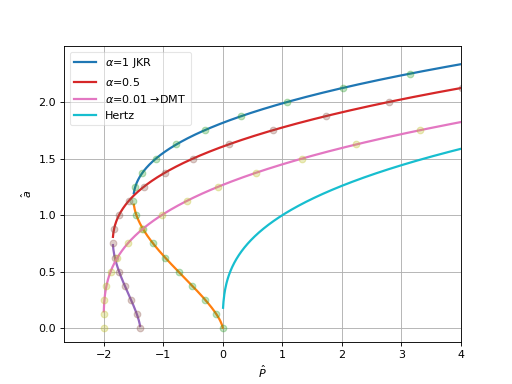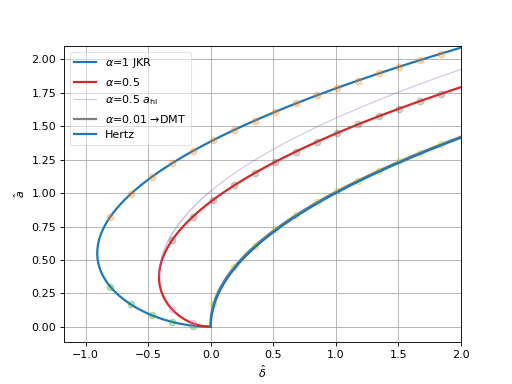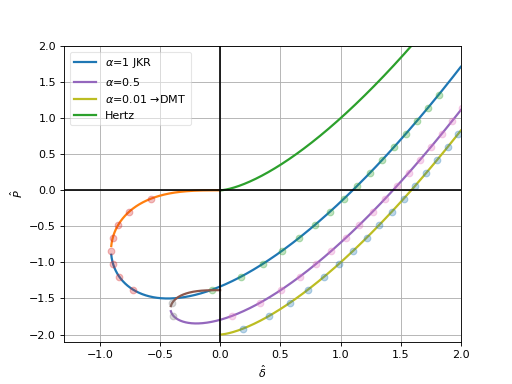In addition to hertzian models, adhesive models capture the effect of distance-dependent attractive force between particles. Adhesive models are usually mathematically rather complicated, extending the Hertz theory in some way. Usually the equations for $$\delta(a)$$ and $$a(P)$$ are provided, reflecting the typical use of adhesive models in microscopy fields. For DEM models, the relationship $$P(\delta)$$ is needed. In some cases, the $$\delta(a(P))$$ is not analytically invertible and solution must be sought by iteration which can have some performance impacts.

Symbols used for adhesive models, in addition to those introduced for Hertzian models) are:

 $$\gamma$$ surface energy (in models with adhesion) $$P_{na}$$ normal adhesive (also called “pull-off” or “sticking”) force $$P_c$$ critical force (leads to surface separation); maximum tensile force an adhesive contact can sustain $$\alpha$$ [COS99] transition parameter between DMT and JKR models $$\hat\delta$$ reduced penetration (dimensionless) $$\hat P$$ reduced total normal force (dimensionless) $$\hat a$$ reduced contact radius (dimensionless)

[Mau92] introduced normalized (dimensionless) numbers of some quantities on adhesive contacts; they are useful for validating models against published plots regardless of the value of input parameters. These units are defined as follows:

\begin{align*} \hat a&=a\left(\frac{K}{\pi\gamma R^2}\right)^{\frac{1}{3}}, \\ \hat\delta&=\delta\left(\frac{K^2}{\pi^2\gamma^2 R}\right)^{\frac{1}{3}}, \\ \hat P&=P\frac{1}{\pi\gamma R}. \end{align*}

## Derjaugin-Muller-Toporov (DMT)¶

The DMT model superposes adhesion outside of the area of contact as predicted by the Hertz model. The magnitude of adhesion is derived from surface energy $$\gamma$$ using thermodynamic approach ([Mod13] pg 39; [DMT75] derives adhesion force for one surface with nonzero surface energy, here we suppose they have together the surface energy of $$2\gamma$$):

$P_{na}=-4\pi R\gamma$

which acts against (negative sign) the elastic repulsion of particles (eq); the total normal force then reads $$P_n=P_{ne}+P_{nv}+P_{na}$$. Contact radius $$a$$ can be expressed from (eq) by replacing $$P_{ne}$$ with total normal force $$P_n$$.

Todo

For completeness mention JKR model; mention Maugis model as the one which accounts for the transition between both, COS introducing the $$\alpha$$ parameter, Schwarz (below) giving analytical solution.

## Schwarz model¶

Schwarz [Sch03] proposes model which accounts for the DMT-JKR transition and, unlike the Maugis “Dugdale” model ([Mau92]), gives the $$a(F)$$ and $$\delta(a)$$ analytically. The surface energy is decomposed as short-range adhesion $$w_1$$ and long-range adhesion $$w_2$$, i.e.

(9)$\gamma=w_1+w_2$

and their proportion determines whether the model is DMT-like or JKR-like. The critical load is superposition of critical forces found in the JKR and DMT models respectively ([Sch03] (23)):

(10)$P_c=-\frac{3}{2}\pi R w_1-2\pi R w_2.$

### DMT-JKR transition¶

For convenience, we will use the $$\alpha\in\langle 0\dots 1\rangle$$ dimensionless parameter introduced by Carpick, Ogletree and Salmeron in [COS99] defined as ([Sch03] (34))

$\alpha^2=-\frac{3 P_c+6\pi R \gamma}{P_c}$

and by rearranging

(11)$P_c=-\frac{6\pi R \gamma}{\alpha^2+3}.$

Combining (9), (10) and (11) leads to

$\gamma\left(\frac{-12}{\alpha^2+3}+4\right)=w_1$

and by further rearrangements we can relate the ratio of short-range and long-range adhesion forces to $$\alpha$$ both ways:

\begin{align}\begin{aligned}\frac{w_1}{w_2}&=\frac{3(1-\alpha^2)}{4\alpha^2}\\\alpha^2=\frac{3}{4\frac{w_1}{w_2}+3}\end{aligned}\end{align}

We can see that extreme values of $$\alpha$$ recover DMT (as limit) or JKR models, and intermediate values represent transition between them:

 $$\alpha$$ $$w_1$$ $$w_2$$ model → 0 → 0 $$\gamma$$ → DMT 1 $$\gamma$$ 0 JKR

Contact radius and force are related by the function ([Sch03] (36))

$a=\left(\frac{R}{K}\right)^{\frac{1}{3}}\left(\alpha\sqrt{-P_c}\pm\sqrt{P_n-P_c}\right)^{\frac{2}{3}}$

which is analytically invertible to

$P_n=\left(\sqrt{a^3\frac{K}{R}}-\alpha\sqrt{-P_c}\right)^2+P_c.$

The following plot shows both functions (dots are the inverse relationship; this plot appears in [Mau92], Fig. 5.):### Peneration¶

Penetration is given as ([Sch03] (27))

(12)$\delta=\frac{a^2}{R}-4\sqrt{\frac{\pi a}{3 K}\left(\frac{P_c}{\pi R}+2\gamma\right)}.$

Plugging (11) into (12), we obtain

(13)$\delta(a)=\frac{a^2}{R}-4\underbrace{\sqrt{\frac{2\pi\gamma}{3K}\left(1-\frac{3}{\alpha^2+3}\right)}}_{\xi}\sqrt{a}=\frac{a^2}{R}-4\xi\sqrt{a}$

where the $$\xi$$ term was introduced for readability. This equation is not analytically invertible and has to be solved numerically. We can find the global minimum as

\begin{align*} \delta'(a)&=\frac{2a}{R}-2\xi a^{-\frac{1}{2}} \\ \delta'(a_{\min})&=0 \\ a_{\min}&=(\xi R)^{\frac{2}{3}} \\ \delta_{\min}&=\delta(a_{\min})=-3R^{\frac{1}{3}}\xi^{\frac{4}{3}}. \end{align*}

The second derivative

$\delta''(a)=\underbrace{\frac{2}{R}}_{>0}+\underbrace{\xi a^{-\frac{3}{2}}}_{\geq 0}>0$

is strictly positive as $$\xi$$, $$R$$ and positive and $$a$$ non-negative.

Given known penetration $$\delta$$, we can find the corresponding value of $$a$$ with Newton-Raphson or Halley’s methods. There are two solutions for all $$\delta\in(\delta_{\min}\dots 0\rangle$$. The solution for the ascending branch ($$\delta'(a<a_{\min})>0$$) is energetically unstable and we can ignore it in dynamic simulations.

As the initial solution for iteration, we can use the (stable) value for zero overlap (satisfying $$\delta(a_0)=0$$) expressed from (13) as $$a_0=(4\xi R)^{\frac{2}{3}}$$ for fresh contacts (where the overlap is likely close to zero); for previously existing contacts, the previous value of $$a$$ is a good initial guess.

This plot shows both loading and unloading (unstable) branches, obtained via Newton iteration (bisection for the unstable branch for simplicity); this plot reproduces [Mau92], Fig. 6.:Note

Values bracketing possible solutions, $$a\in\langle a_{\mathrm{lo}}, a_{\mathrm{hi}}\rangle$$ (lower and upper brackets) are to be defined for guarded iterative methods.

The lower bracket is

$\begin{split}a_{\mathrm{lo}}=\begin{cases} a_{\min}=(\xi R)^\frac{2}{3} & \text{if \delta<0} \\ a_0=(4\xi R)^{\frac{2}{3}}=4^\frac{2}{3}a_{\min} & \text{if \delta\geq0} \end{cases}.\end{split}$

The upper bracket $$a_{\mathrm{hi}}$$ can be obtained from (13). Since $$\delta'(a)$$ is bounded by

(14)$\delta'(a)=\frac{2a}{R}-\underbrace{2\xi a^{-\frac{1}{2}}}_{\geq0}\leq\frac{2a}{R}=\delta_{\mathrm{Hertz}}'(a)$

where $$\delta_{\mathrm{Hertz}}=a^2 R$$ is the Hertz limit valid for $$\alpha\to0$$; it is a purely geometrical relationship, valid also for the DMT model. It follows for the stable branch of $$a(\delta)$$ that

$\delta(a>a_{\min})\geq \delta_{\mathrm{Hertz}}(a-a_{\min})+\delta_{\min},$

i.e. that $$\delta_{\mathrm{Hertz}}(a)$$ with the origin shifted to $$(a_{\min},\delta_{\min})$$ is the lower bracket for $$\delta(a)$$. Since $$\delta'(a)=1/a'(\delta)$$ (as per derivative rule for inverses), the inequality (14) implies

$a(\delta)\leq a_{\mathrm{hi}} = a_{\mathrm{Hertz}}(\delta-\delta_{\min})+a_{\min}=\sqrt{R(\delta-\delta_{\min})}+a_{\min}.$

The upper bracket is shown in the plot above for $$\alpha=0.5$$.

By composing $$P_n(a)$$ and (numerically evaluated) $$a(\delta)$$, we obtain the displacement-force relationship ([Mau92], Fig. 7.)Implementation note:

DMT/Schwarz contact models can be used via woo.dem.Law2_L6Geom_HertzPhys_DMT, when woo.dem.Cp2_FrictMat_HertzPhys.gamma (surface energy $$\gamma$$) is non-zero; woo.dem.Cp2_FrictMat_HertzPhys.alpha (Carpick-Ogletree-Salmeron transition parameter $$\alpha$$) determines the model behavior on the continuous DMT ($$\alpha=0$$) – JKR ($$\alpha=1$$) range. Appropriate engines are returned from woo.dem.DemField.minimalEngines when model is an instance of woo.models.ContactModelSelector with name equal to "DMT" or "Schwarz".

Tip

Report issues or inclarities to github.